## Speed Torque Characteristics of DC Series Motor:

To find the Speed Torque Characteristics of DC Series Motor, let us analysis the figure 7.43 shows the connection diagram of a dc series motor. The speed for this motor is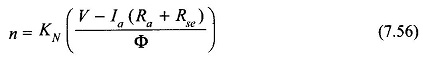and the torque equation is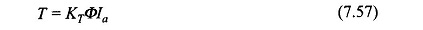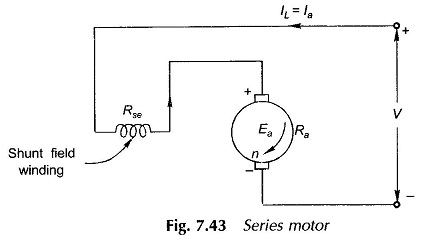Consider at first the case of linear magnetization, i.e,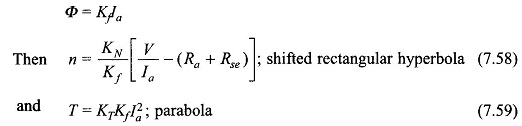These relationships are plotted in Figs 7.44(a) and (b). Saturation and armature reaction demagnetization both cause the flux/pole to increase (with respect to Ia) at a rate slower than in the assumed linear relationship. Actual characteristics are plotted in dotted lines in Fig. 7.44(a) and (b).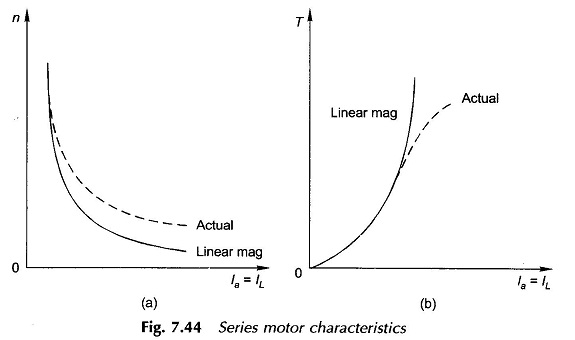It is easily observed that at no-load, the motor current and hence the flux/pole tends to zero and as a consequence (Eq. (7.58)), the motor speed tends to increase to infinity. This is a dangerous situation and the centrifugal forces will destroy the armature. Hence, a series motor must never be allowed to run at no-load even accidentally.

Eliminating Ia from Eq. (7.58) with the help of Eq. (7.59), the Speed Torque Characteristics of DC Series Motor is obtained as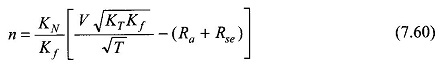which is plotted in Fig. 7.45. It is observed that as the load torque increases the motor speed drops heavily relieving thereby the kW load (n x T) on the motor. This type of speed-torque characteristic is known as the “series” characteristic and is ideally suited for traction, cranes, etc.—applications wherein during starting a large accelerating torque is demanded by the load. In the two applications cited, the load is always on the motor so there is no danger of under-loading or no-loading.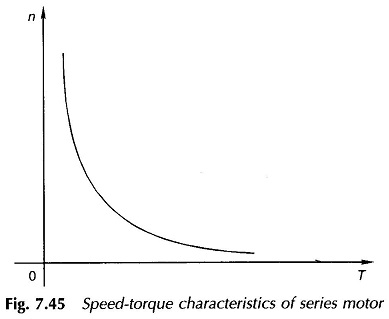Scroll to Top